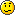# what is the use of AND/XOR ?!

Member Posts: 48 ■■□□□□□□□□
Hello everyone,

I want to know what is the use of AND/XOR in wildcard masks?:

Is it for summarizing ip addresses the most efficient way?:

Am i getting it right?

• Basically AND operation can be used to compare binary numbers.

About 20 or more years ago, I studied Assembly Language. This was before routers or IPs. As you know, Computers are based on 1s and 0s. It would be redundant to change 8 bits to a decimal number to compare, so, it would be easier to just do an AND function to find if there is no difference between two numbers.

I know for certain the operations are used in comparing one number to another. Also, because I didn't learn Assembly language fully, I'm not 100% certain, but AND, Or, XOR operations might be used to add, subtract, binary numbers by moving bits to the left or right.
• Member Posts: 1,057 ■■■■■■□□□□
Another way to think about AND/XOR is the way your brain logic works. AND to compile a list of two or more things where you need them all. XOR when you want to determine if two or more things are different but you don't care initially which are different. i.e. one of these things are not like the other when you were in primary school. OR when you just want to have something from a list of like items. i.e. you walk into a bar and say give me a beer.Oh and @Avocado simple add and subtracts in assembly generally use shift left and shift right operators for speed.
Who knows what evil lurks in the heart of technology?... The Shadow DO
• Member Posts: 1,340 ■■■■□□□□□□
As previously stated, AND can be used for comparison or to find the state of a specific bit in a byte or word among other things. XOR can be used for very weak encryption (Isn't this what Cisco uses when you use the enable password command) or to exchange the value of two variables/registers without using an intermediate variable/register among other things.

Example of XOR Exchange:

A = A XOR B
B = B XOR A
A = A XOR B

A = AD, B = CE

A = AD XOR CE = 63
B = CE XOR 63 = AD
A = 63 XOR AD = CE

A = CE, B = AD

Let's say you have the number 10 (A in hexadecimal) and wanted to know whether a certain bit was a 1 or 0, you could

A AND (2^N)

For example...

A AND (2^0) = 0
A AND (2^1) = 2
A AND (2^2) = 0
A AND (2^3) = 8

If the result is non-zero then the bit is a one. In this case A (Hexadecimal) is 1010 (Binary).
R&S: CCENT CCNA CCNP CCIE [ ]
Security: CCNA [ ]
Virtualization: VCA-DCV [ ]